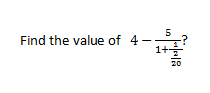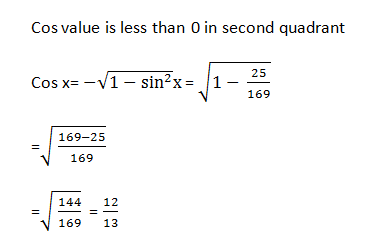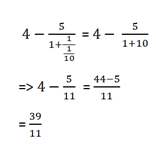# SSC CGL 2018 Practice Test Papers | Quantitative Aptitude (Day-21)

Dear Aspirants, Here we have given the Important SSC CGL Exam 2018 Practice Test Papers. Candidates those who are preparing for SSC CGL 2018 can practice these questions to get more confidence to Crack SSC CGL 2018 Examination.

[WpProQuiz 2971]

Click “Start Quiz” to attend these Questions and view Explanation

1)  Two numbers X and Y are in the ratio 5:6.  If 40% of first number is equal to one third of second number. Find the value of X.

a) 60

b) 50

c) 72

d) Can’t be determined

2) For any two angles which have one common side and the sum of the two angles is supplementary then they are called as

b) Collinear angles

c) Corresponding angles

d) Linear Pair

3) if sin x=5/13, 90<x<180. Find the value of cos x.

a) -12/13

b) 12/13

c) 0

d) -5/13

4) Hridhaya’s salary is 50% more than Ananya’s salary.  If Hridhaya saves Rs.1200 per month which is 40% of Ananya’s salary. Find Hridhaya’s salary.

a) 10000

b) 5000

c) 4500

d) 7000

5) The average of 15 consecutive odd natural numbers starting from 333 to 361 is x. Find the average if 4 more consecutive odd natural numbers are added before 333.

a) 350

b) 347

c) 343

d) 351

6).a) 40/13

b) 39/11

c) 49/15

d) 20/11

7) If a3-b3 =45 and a-b=3 then find a2+b2?

a) 15

b) 11

c) 13

d) 20

8) Pipes A and B can fill the tank in 15 and 20 hours. Pipe C is an outlet which can empty the tank in 30 hours. All three pipes are opened simultaneously and pipe C is closed after 5 hours. What part of the tank is yet to be filled?

a) 5 /12

b) 6/11

c) 7/12

d) 4/12

9) The perimeter of a rectangle and square are equal and is 200 m. The area of square is 100 m2 more than area of rectangle. What is the difference between Length of rectangle and side of a square?

a) 10 m

b) 20 m

c) 25 m

d) 30 m

10) By selling a camera at a profit of 30% the shopkeeper got Rs. 625 more than one-third of the cost price. Find the cost price.

a) 1250

b) 2000

c) 3500

d) 4000

x/y=5/6— (i)

40x/100=y/3

=>x/y=5/6—- (ii)

Both equations are same.

So the value of x cannot be determined.

For any two angles which have one common side and the sum of the two angles is supplementary then they are called as Linear Pair.40 % of ananya salary =1200

100 % of ananya salary= 1200×100/40=3000

Hridhaya salary = 3000x 150/100=4500

Average of numbers from 333 to 361= (333+361)/2=347

Avg of all odd natural numbers if 4 numbers are added before 333= 347-4=343.a2+b2= (a-b)2+2ab —– (i)

a3-b3= (a-b) (a2+ab+b2) —— (ii)

Substitute (i) in (ii)

a3-b3= (a-b) ((a-b)2+2ab +ab)

45 = 3(9+3ab)

15=9+3ab

sb = 2—– (iii)

Sub (iii) in (i)

a2+b2= 32+2×2=13

A=1/15

B=1/20

C=-1/30

Part of tank filled in 5 hours= 5(1/15+1/20 – 1/30) = 5(5/60) = 25/60 = 5/12

Remaining part to be filled = 7/12

Perimeter of a rectangle = 2 (l+b)

Perimeter of a square = 4a = 200

a=50

2(l+b) = 4a

2(l+b) = 200

(l+b) = 100—– (i)

Area of square= a^2=2500

Area of Rectangle= lb

a2-lb=100

2500-lb=100

Lb = 2400——– (ii)

On solving (i) and (ii)

L=60

l-a=60-50=10

13/10-4/5=5/10=1/2

1/2———– 625

1————–?

?= 625 x2=1250